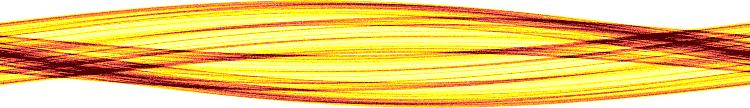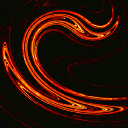Research Interests

In the beginning, how the heav'ns and earth rose out of chaos - John Milton.

In this section we briefly explained many of our theoretical research interests and, if possible, we provide some references in the general science literature. For works of a more technical nature please refer to the papers section or click the appropriate paper icon below for the topic of your choice. For a brief overview of chaos we refer you to

• C. Grebogi, E. Ott, and J.A. Yorke, "Chaos, Strange Attractors, and Fractal Basin Boundaries in Nonlinear Dynamical Systems", Science, 238, 585 (1987).
For a more extensive treatment see the book

The following list should come in handy for navigational purposes:

## Chaotic Scattering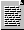In the classical dynamics potential scattering problem one considers a Hamiltonian H = p/2m + V(r), where the potential V approaches zero for large r (the magnitude of r) . One then asks how outgoing orbits at large r depend on incoming orbits. For example, one might plot scattering angle as a function of impact parameter. In typical cases, such functions can have exceedingly complex behavior, where the function is singular on a fractal (uncountable) set of impact parameter values. This type of behavior is indicative of the presence of an unstable chaotic set in the dynamics.

• Maddox, John, "Is Chaos becoming conversational?", Nature, 341, No. 7, pg. 17, Sept. 1989.

## Chaotic Transients

In chaotic transients one observes that typical initial conditions initially behave in an apparently chaotic manner for a possibly long time, but, after a while, then rapidly move off to some other region of phase space, perhaps asymptotically approaching a nonchaotic attractor. The length of such chaotic transients depends sensitively on initial conditions and exhibits a characteristic Poisson distribution for randomly chosen initial conditions.

## Communicating with Chaos

Much of the fundamental understanding of chaotic dynamics involves concepts from information theory, a field developed primarily in the context of practical communication. Concepts from information theory used in the chaos includes metric entropy, topologoical entropy, Markov partitions, and symbolic dynamics. It is possible to use the close connection between the theory of chaotic systems and information theory in a way that is more than purely formal. In particular, it can be shown that the recent realization of chaos can be controlled with small perturbations can be utilized to cause the symbolic dynamics of a chaotic system to track a prescribed symbol sequence thus allowing the encoding of any desired message in the signal from a chaotic oscillator. The natural complexity of chaos thus provides a vehicle for information transmission in the usual sense.

## Control and TargetingTwo fundamental aspects of chaos are the following:
1. The exponential sensitivity of orbits to small perturbations.
2. A very complex orbit structure making possible many very different motions in the same system.
Actually these two are not independent; they may be viewed as two sides of the same "chaos coin". The first property leads to the diffculty in the prediction of sufficiently distant future states, while the second property leads to the fact that chaotic attractors often have embedded within them an infinite, dense set of unstable periodic orbits. While chaos is commonly viewed as undesirable, these properties can also be of great benefit in certain situations where one wishes to control the system dynamics. In particular, if the system is sufficiently well characterized and noise is not too large, then the first property implies that relatively large changes in the eventual location of orbit points can be made using only small changes in a control variable, while the second property implies that the dynamical changes so produced may be very diverse, leading to a situation with a great deal of flexibility. The two methods that are useful in utilizing chaos in an effective ways are: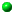Control
Feedback stabilization of selected unstable orbits embedded in the attractor.Targeting
The rapid steering of a chaotic orbit to the vicinity of a desired point in phase space.

• T. Shinbrot, C. Grebogi, E. Ott, and J.A. Yorke, "Using Small Perturbations to Control Chaos", Nature, 363, 411 (1993).
• Peterson, Ivars, "Ribbon of Chaos" Science News, 139, No. 4, pg. 60, Jan. 26, 1991.
• Langreth, Robert, "Engineering Dogma gives way to Chaos", Science, 252, pg. 776, May 1991.
• Corcoran, Elizabeth, "Ordering Chaos", Scientific American, pg. 96, Aug. 1991.

## CrisesAnother type of transition to a chaotic attractor is the crises. Basically, what happens in this case is that the unstable chaotic set responsible for a chaotic transient becomes stable as the parameter p is increased through a critical crisis value p. When it becomes stable, the chaotic set formerly responsible for the chaotic transient becomes a chaotic attractor. For parameter values in the transient range, p > p, there is typically a characteristic dependence of the mean duration of chaotic transients on p. Namely,(p-p), where the critical exponent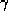can be obtained from a knowledge of the instability properties of certain unstable periodic orbits on the chaotic set. This dependence ofon p makes clear the nature of the transition: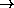as ppfrom below, thus converting the transient to long-term time-asymptotic behavior.

## DimensionsPerhaps the most basic aspect of an attractor is its dimension. While it is clear that the dimension of a fixed point in phase space is zero and that of the limit cycle is one, it is also the case that invariant sets arising in dynamical systems (such as chaotic attractors) often have structure on arbitrarily fine scale, and the determination of the dimension of such sets is nontrivial. In such cases the assignment of a dimension value gives a much needed quantitative characterization of the geometrical structure of a complicated object.

## Fast Magnetic DynamosMagnetic fields are pervasive in the Universe. A natural approach to explain their prevalence is the kinematic dynamo problem: Given a flow of a conducting fluid, will a small seed magnetic field amplify exponentially with time? If the answer is yes then the zero magnetic field state is unnatural, and the flow will self-generate its own magnetic field. Recently it has been shown that chaos in the Lagrangian dynamics of the underlying flow is the key consideration for answering the question posed by the kinematic dynamo problem.

## Fractal Basin BoundariesBasin boundaries arise in dissipative dynamical systems when two, or more, attractors are present. In such situations each attractor has a basin of initial conditions which lead asymptotically to that attractor. The basin boundaries are the sets which separate different basins. It is very common for basin boundaries to contain unstable chaotic sets. In such cases the basin boundaries can have very complicated fractal structure. Because of this complicated very fine-scaled structure, fractal basin boundaries can pose an impediment to predicting long-term behavior. In particular, if an initial condition is specified with only finite precision, it may be very difficult a priori to determine in which basin it lies if the boundaries are fractal.

## NetworksThe interconnection of large numbers of dynamical units is common across a variety of fields including such applications as the internet, the world wide web, gene networks, and the web of social interactions between people, corporations, and animals. Recent research in this area has focused on characterizing network properties, on the evolution and growth of networks, and on the relation of network topology to the dynamics and functioning of networked systems.

## Pattern FormationPattern formation in non-equilibrium systems has drawn intensive attention and has been the subject of much rigorous investigation in recent years. Belousov-Zhabontinskii(BZ) chemical reaction, colonies of social amoebaem, electical excitation propagation in the heart are situations where considerations of spatiotemporal pattern dynamics are important. As the simplest description of such systems, we have investigated various aspects of the Complex Ginzburg Landau Equation, especially the formation of spiral waves.

## Prediction

One of the fundamental characteristics of chaotic processes is the failure of long-term predictability. Unlike nonchaotic cases, where the system settles into an equilibrium or regular oscillatory mode, a system whose evolution is described by a chaotic attractor exhibits many of the properties of a random process. In fact, this superficial resemblance of a chaotic process to a random process was one of the reasons that chaos was not generally recognized in practice until recent years. Systems that entered a regime in which they oscillated irregularly were equated with random processes, and therefore considered unpredictable except in a statistical way. More recently methods of short-term prediction of chaotic processes have been formulated. These methods make use of the ergodicity of orbits on chaotic attractors. In particular, ergodicity implies the ability to learn dynamics from observations of the system and then use that knowledge for prediction.

## Quantum ChaosAccording to the correspondence principle, there is a limit where classical behavior as described by Hamilton's equations becomes similar, in some suitable sense, to quantum behavior as described by the appropriate wave equation. Formally, one can take this limit to be h approaching zero, where h is Planck's constant; alternatively, one can look at successively higher energy levels, etc. Such limits are referred to as "semiclassical". It has been found that the semiclassical limit can be highly nontrivial when the classical problem is chaotic. The study of how quantum systems, whose classical counterparts are chaotic, behave in the semiclassical limit has been called quantum chaos. More generally, these considerations also apply to elliptic partial differential equations that are physically unrelated to quantum considerations. For example, the same questions arise in relating classical acoustic waves to their corresponding ray equations. Among recent results in quantum chaos is a prediction relating the chaos in the classical problem to the statistics of energy-level spacings in the semiclassical quantum regime.

## Riddled BasinsThe notion of determinism in classical dynamics has eroded since Poincaré's work led to recognition that dynamical systems can exhibit chaos: small perturbations grow exponentially fast. Hence, physically ubiquitous measurement errors, noise, and computer roundoff strongly limit the time over which, given an initial condition, one can predict the detailed state of a chaotic system. Practically speaking, such systems are nondeterministic. Notwithstanding the quantitative uncertainty caused by perturbations, the system state is confined in phase space (on an "attractor") so at least its qualitative behavior is predictable. Another challenge to determinism arises when systems have competing attractors. With a boundary (possibly geometrically convoluted ) between sets of initial conditions tending to distinct attractors ("basins of attraction"), perturbations make it difficult to determine the fate of initial conditions near the boundary. Recently, mathematical mappings were found that are still worse: an attractor's entire basin is riddled with holes on arbitrarily fine scales. Here, perturbations globally render even qualitative outcomes uncertain; experiments lose reproducibility.

• J.C. Sommerer and E. Ott, "A Qualitatively Nondeterministic Physical System", Nature, 365, 135 (1993).

## ShadowingChaotic processes have the property that relatively small numerical errors tend to grow exponentially fast. In an iterated process, if errors double each iterate and numerical calculations have 48-bit (or 15-digit) accuracy, a true orbit through a point can be expected to have no correlation with a numerical orbit after 50 iterates. One may therefore question the validity of a computer study over times longer than this relatively short time. A relevant result in this regard is that of Anosov and Bowen who showed that systems which are uniformly hyperbolic will have the shadowing property: a numerical (or noisy) orbit will stay close to (shadow) a true orbit for all time. Unfortunately, chaotic processes typically studied do not have the requisite uniform hyperbolicity, and the Anosov-Bowen result does not apply. Recent results of our group apply to nonhyperbolic situations.

• Cipra, Barry A., "Computer-Drawn Pictures Stalk the Wild Trajectory", Science, 241 pg. 1162, Sept. 1988.
• Peterson, Ivars, "In the Shadow of Chaos", Science News, 134, No. 23, pg. 360, Dec. 3, 1988.
• Maddox, John, "How to shadow noisy chaos", Nature, 347, No. 18, pg. 613, Oct. 1989.

## Unstable Chaotic Sets

Attractors refer to sets which "attract" orbits and hence determine typical long-term behavior. It is also possible to have sets in phase space on which the dynamics can be exceedingly complicated, but which are not attracting. In such cases orbits placed exactly on the set stay there forever, but typical neighboring orbits eventually leave the neighborhood of the set, never to return. One indication of the possibility of complex behavior on such nonattracting (unstable) sets is the presence within these sets of periodic orbits whose number increases exponentially with their period, as well as the presence of an uncountable number of nonperiodic orbits. Nonattracting unstable chaotic sets can have important observable macroscopic consequences. Three such consequences are the phenomena of chaotic transients, fractal basin boundaries, and chaotic scattering.